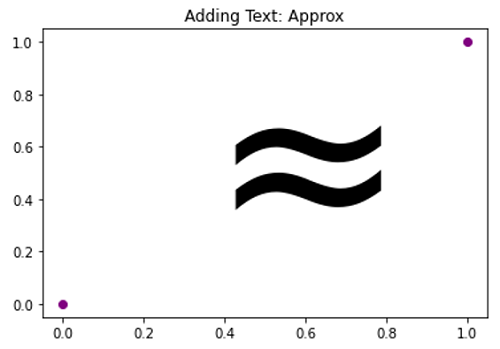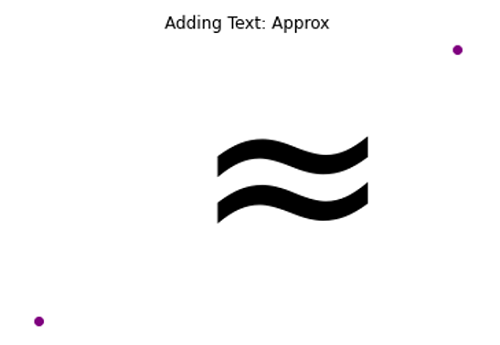# Approx Symbol in Python Plotting

Here, we are going to learn how to add approx symbol in Python plotting?
Submitted by Anuj Singh, on September 11, 2020

Approx a different symbol used in set theory and other mathematical expressions. Python has provided us to use it while plotting. Following example shows its implementation.

```plt.text(0.25, 0.25, r'\$\approx\$', fontsize=175)
```

Illustrations:## Python code for Approx symbol in plotting

```import numpy as np
import matplotlib.pyplot as plt

# Approx
# In text
plt.figure()
plt.plot([0,1],[0,1], 'o', color=  'purple')
plt.text(0.25, 0.25, r'\$\approx\$', fontsize=175, )
plt.show()

plt.figure()
plt.plot([0,1],[0,1], 'o', color=  'purple')
plt.text(0.25, 0.25, r'\$\approx\$', fontsize=175, )
plt.axis(False)
plt.show()
```

Output:

```Output is as Figure
```

Languages: » C » C++ » C++ STL » Java » Data Structure » C#.Net » Android » Kotlin » SQL
Web Technologies: » PHP » Python » JavaScript » CSS » Ajax » Node.js » Web programming/HTML
Solved programs: » C » C++ » DS » Java » C#
Aptitude que. & ans.: » C » C++ » Java » DBMS
Interview que. & ans.: » C » Embedded C » Java » SEO » HR
CS Subjects: » CS Basics » O.S. » Networks » DBMS » Embedded Systems » Cloud Computing
» Machine learning » CS Organizations » Linux » DOS
More: » Articles » Puzzles » News/Updates